#DAY 30
2
AI & Data

## 前言

AutoML 想要滿足普羅大眾的夢想，不用學習一堆的演算法，只要你指定工作類別，它就幫你找到最佳模型及最佳參數，當然包括預測結果，哇，這麼神，不一探究竟怎麼可以。

AutoKeras 就是以 Keras 風格撰寫的 AutoML 套件，目前提供三類功能：

1. 影像分類與迴歸(Image Classification and Regression)
2. 文字分類與迴歸(Text Classification and Regression)
3. 結構化資料分類與迴歸(Structured Data Classification and Regression)：即一般的表格資料，如 CSV、Excel、資料庫...等二維表格資料。

AutoKeras 撰寫非常簡單，我們就來玩玩看吧。

## 讓我們開始吧

1. 安裝很簡單，執行下列指令即可：
``````pip install git+https://github.com/keras-team/keras-tuner.git@1.0.2rc2
pip install autokeras
``````
1. 簡單測試【手寫阿拉伯數字】辨識。
• 複製第三篇範例 03_01_MNIST.ipynb，另存為 30_01_AutoKeras_MNIST.ipynb。
• 載入MNIST資料集後，特徵縮放、One-hot encoding、指定損失函數、優化器、效能衡量指標通通都不需要，直接訓練即可，程式碼如下：
``````import tensorflow as tf
mnist = tf.keras.datasets.mnist

# 匯入 MNIST 手寫阿拉伯數字 訓練資料
(x_train, y_train),(x_test, y_test) = mnist.load_data()

import autokeras as ak

# 初始化影像分類器(image classifier)
clf = ak.ImageClassifier(
overwrite=True,
max_trials=1)
# 訓練模型
clf.fit(x_train, y_train, epochs=10)
``````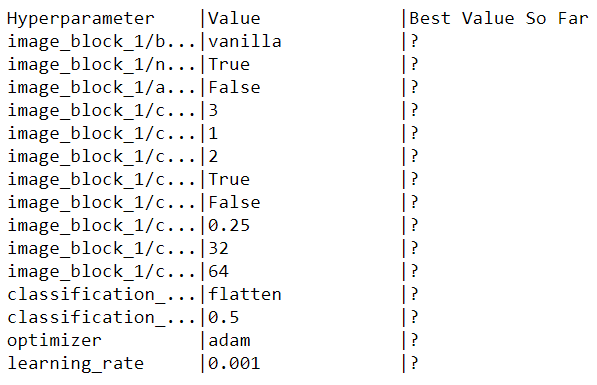• 評估：準確度也很高，連很潦草的第9筆也辨識正確。
``````# 預測
predicted_y = model.predict(x_test)

# 評估，打分數
print(model.evaluate(x_test, y_test))
``````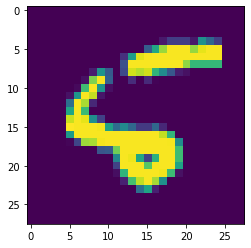• 使用自己寫的數字測試一下，哇，全對。
``````# 使用小畫家，寫0~9，實際測試看看
from skimage import io
from skimage.transform import resize
import numpy as np

X_ALL = np.empty((0, 28, 28))
for i in range(10):
image1 = io.imread(f'./myDigits/{i}.png', as_gray=True)
#image1 = Image.open(uploaded_file).convert('LA')
image_resized = resize(image1, (28, 28), anti_aliasing=True)
X1 = image_resized.reshape(1, 28, 28) #/ 255
# 反轉顏色
# 顏色0為白色，與RGB顏色不同，(0,0,0) 為黑色。
# 還原特徵縮放
X1 = (np.abs(1-X1) * 255).astype(int)
X_ALL = np.concatenate([X_ALL, X1])
predictions = model.predict(X_ALL)
print(predictions)
``````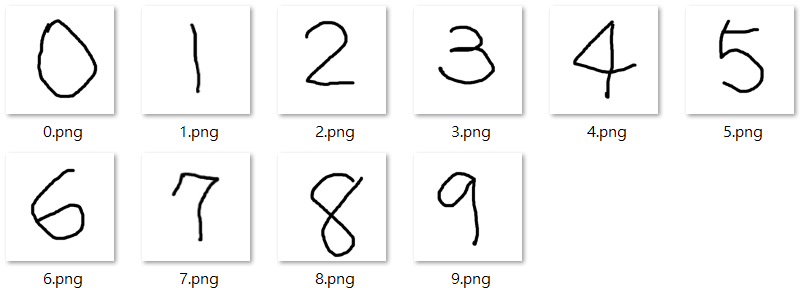## Fashion MNIST 辨識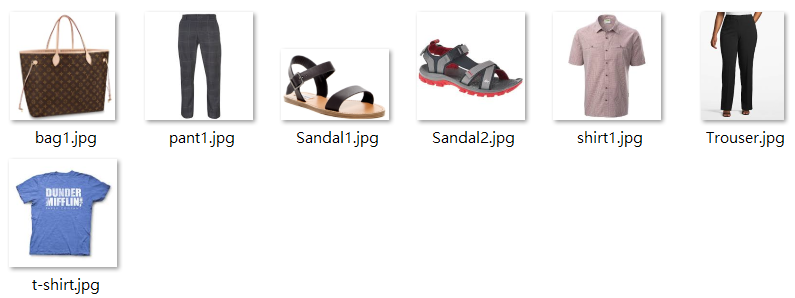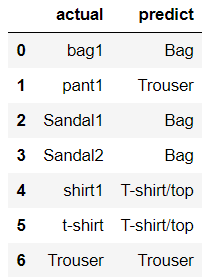## 結論

AI只會取代不懂AI的員工，懂得運用AI的員工永遠不會被取代。